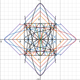Unresolved Detail In Plotted Equations

Sometimes the calculator detects that an equation is too complicated to plot perfectly in a reasonable amount of time. When this happens, the equation is plotted at lower resolution.

216 out of 241 found this helpful
Have more questions? Submit a request

•Tanksear Industries

\csc\left(\frac{\sin\left(x!y\right)}{xy\cdot\sqrt{yyx}}\right)\ge x^0

•Tanksear Industries

\operatorname{median}\left(\sin\left(\operatorname{mod}\left(x,y\right)\right)\sin\left(y!\right)\cos\left(x!^2\right),\sqrt{\sqrt{\left|\frac{x}{yyx!!\cdot\frac{x}{yy}}\right|}},yxxxyyxyxy,x,\frac{yy!}{xx!}\right)\le\operatorname{mod}\left(\sin x,\cos y\right)

This one is just simply insane.

•Tanksear Industries

https://www.desmos.com/calculator/porvrrhfzb

It doesn't have unresolved detail, but if you go to the right even a little bit, it gets weird. Why is this?

•D35M05BR34K4G3MA5T3R

Just sit back and eat fatty Doritos and see DESMOS BREAKAGE unfold in front of your eyes.  So much heck will tick you off - So I suggest you either see a mental therapist before you see heck, or you see a MENTAL therapist and a doctor because you got an aneurysm from the graph.  Good luck living!

•D35M05BR34K4G3MA5T3R

BTW, none of the non-functions are showing, so show the ones you want to see.

•Tanksear Industries
•Jacob Kinnoin

https://www.desmos.com/calculator/72scyk26ag

i took all of your suggestions and put it into a graph hahahahahahahaha

•Tanksear Industries

\tan\left(\sqrt{\frac{\sin\pi x}{4\pi x}}\left(xx^{\sqrt{\frac{x}{y}}}\right)\right)=0

I'm making weird graphs instead of learning algebra right now

•168673
•Pulsar

a collection of a few zany ones. have fun!

https://www.desmos.com/calculator/sazf5e5gya

•Tanksear Industries

\frac{x}{y}\ge\sin\left(x-y\right)x

This one is pretty interesting
•Tanksear Industries
https://www.desmos.com/calculator/hmksg90dhmDoes anyone else notice the weird sort of gradient in one of the triangles?
•Tanksear Industries
•Tanksear Industries

x+y=x^3+y^u

But you have to either switch a couple exponents or change u.

•Tanksear Industries

\operatorname{mean}\left(x,y,x^2,y^2,\sin\left(x\right),\cos\left(y\right),y+2,y^2,\sin\left(y\right),\tan\left(x+y\right),x\cdot y\right)=y!x+\sin x

I found this while digging around in my graphs

•Tanksear Industries

https://www.desmos.com/calculator/sxplv8onxi

Y'all get the joke...?
•David Robillard

\frac{\sin\left(x!\right)+\sqrt[y]{x!}}{\tan\left(x^y\right)}\cdot\frac{\cot\left(y^x\right)}{\cos\left(y!\right)-\sqrt[x]{y!}}=\frac{\frac{\sec\left(y!\right)-\sqrt[x]{y!}}{\cot\left(x^y\right)}}{\frac{\tan\left(y^x\right)}{\csc\left(x!\right)+\sqrt[y]{x!}}}

•Janders2

\operatorname{mod}\left(y,2\right)=\frac{\left(\sec\left(x\right)+3\right)}{e+\sin\left(5xy\right)}

This is insanity.

•Jase W Andersonyoung

\frac{\left(xy\cdot\ln\left(2\left(x^2\right)\right)\right)}{\cos\left(\sin\left(e\right)\right)}=\frac{yx}{e}+\frac{\left(\frac{\left(\frac{\left(\frac{\sin b}{\pi}+3\left(\tan\left(y\right)-\ln\left(y\right)\right)^{\left(3+2x\right)}-\frac{6x^2}{7y}\right)}{\sqrt{2^{\frac{x}{2}}}-\frac{\left(8+x\cdot2\operatorname{mod}\left(4e,2y\right)\right)}{8-\sin y}}\right)}{\frac{\left(\cos\left(\tan\left(y\right)+\sec\left(\frac{6x}{y}\right)\right)\right)}{\cot\left(6y\right)+2^a}-\frac{\left(\sin x+\sin y\right)}{\tan y+\ln x-\sin\left(x^2\right)}}+\frac{\left(\frac{\ln6}{y}+\cos x\right)}{\sum_{n=\frac{5}{x}}^{\sin\left(\sqrt{yx}\right)}\ln\left(\sin\left(\frac{x}{3y}\right)\right)}\right)}{\left(\sqrt{\operatorname{mod}\left(\sum_{n=y+\frac{\cos x}{\sec\left(y^3\right)}-\ln\left(\frac{3y}{5}\right)}^{3^{\left(x+y\right)}}\frac{5y}{e^x}+\sqrt{3y},6bx^2\cdot y\sin\left(20\right)\right)}\right)}

b=\sqrt{yx}+e^{\sin x^{\left(2\right)}}+6\tan\left(y\right)

a=\frac{\sin x}{\frac{\sin y}{\frac{\sec x}{\pi xy+2}}}

It broke, there's nothing there at all unlike my last one which as i said was insanity.

•300149

y=4x^y

•Tanksear Industries
You will become like the title of this graph.
•Tanksear Industries

https://www.desmos.com/calculator/248moslqhp

The sign of confusion is  \frac{x}{c^{yyx}}\cdot\sqrt{\sqrt{\frac{\frac{x}{y}x}{yyy}}}\cdot\sin\left(x\cdot\sqrt{xy}\right).
Edited by Tanksear Industries
•Tanksear Industries

https://www.desmos.com/calculator/nggzobvv2r

...I should probably be working on my test right now.

•Tanksear Industries

\frac{x}{y}^x\cos\left(\frac{\sqrt{\frac{x}{yy^{\sqrt{yx}}}}xy}{\sqrt{\sqrt{\frac{y}{x^{yyx}-xy}}}}-\operatorname{floor}\left(\frac{yx}{xxy\cdot x^y}\right)\cdot\sqrt{\sqrt{\frac{xy}{x}}}\right)\le\operatorname{ceil}\left(\sin\left(\frac{x}{\sqrt{yx\cdot\sqrt{\sin\left(\sqrt{\sqrt{xx^{\sqrt{y}}}}\right)}}}-\sqrt{\frac{x}{y}\cdot\sqrt{\sqrt{\frac{yyx}{yx}}}}\cdot xxx^{\sqrt{xyx}}\cdot\sqrt{\sqrt{\frac{ex}{x\%\operatorname{of}yyex}}}\right)\right)

for a good spot, https://www.desmos.com/calculator/s1ymshnxvy

•Tanksear Industries

0\le\sin\left(\frac{y}{\sin\left(\frac{x}{\sin\left(\frac{y}{\sin\left(\frac{x}{\left(\sin\left(\frac{y}{\sin\left(x\right)}\right)\right)}\right)}\right)}\right)}\right)

•Tanksear Industries

https://www.desmos.com/calculator/al8k1gmqyd
Try moving around in this one. It's weird.

•Alexander

You can also try \sin y=\frac{x^2}{y^3}, it turns out kinda weird

•Noah Albrecht2000

\left|y\right|\ =\ \sin\left(\frac{x}{3.5}\right)^2\cdot x

ist just getting bigger and it looks cool

•GlitchedGraph666

\frac{\sin\left(x!\cdot y!\right)}{\tan\left(x^2!+y^2!+x^3!!+y^3!!!\right)}=\sin\left(\frac{x!}{y^2}\cdot\frac{y!}{x^2}\right)

•D35M05BR34K4G3MA5T3R